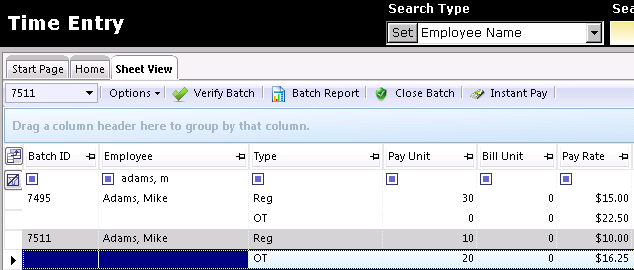# Weighted OT Example

## Overview

Updated 11/16/2021

Weighted OT is required by the Department of Labor (DOL).  If there are multiple transactions for the same employee in the same department, the system automatically weights the OT on the last transaction entered by averaging all of the rates together.

Employee Mike works 60 hours for two different assignments.  He has two different pay rates, so the OT rate must be weighted. However due to regulations we cannot calculate this OT rate by just saying 1.5 x the regular pay rate on the assignment where the OT was accrued.  There are special calculations for this.

Mike worked 30 hours at job A that paid \$15 an hour (reg rate), and 30 hours at job B that paid \$10 an hour (reg rate).

Traditionally the OT pay rate for the \$10 an hour job would be \$15, but since some of his hours were worked at \$15 an hour the OT pay rate is no longer \$15.  Here is how weighted OT calculates:

First total pay must be calculated to determine the calculated reg rate which will determine the OT premium rate needed.

30 HRs @ \$15/HR = \$450.00
30 HRs @ \$10/HR = \$300.00

Total Pay = \$750.00

Calculated Reg Pay Rate = \$750 / 60 = \$12.50 [Total Pay / Total Hours]

OT Premium Rate – The amount you will add to the original Reg Rate on the transaction = \$12.5 * .5 = \$6.25 + Transaction 2 Reg Rate (\$10.00) = \$16.25

Weighted OT Premium: 20 * \$16.25 = \$325 [Number of OT Hours * OT Premium]

Gross Pay Total: \$450 + \$100 + \$325 = \$875.00

## Displayed in Time Entry

Mike

Transaction 1
30 Hrs @ \$15.00 = \$450.00

Transaction 2
10 Hrs @ \$10.00 = \$100.00
20 Hrs @ \$16.25 = \$325.00 [OT Payrate = \$10.00 + (\$750 / 60) * .5)]

Gross Pay = \$875.00Calculation Formula =

 T1 Hours * Reg Rate + T2 Hours * Reg Rate = Reg Wage / Total Hours = Adjusted Reg Rate* 0.5 = OT Factor + T2 Reg Rate (or whichever transaction is having hours added that exceed reg limit) = OT Rate